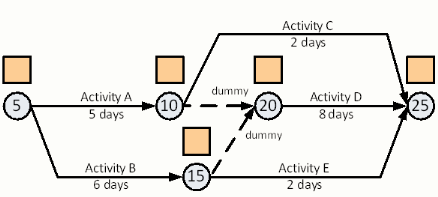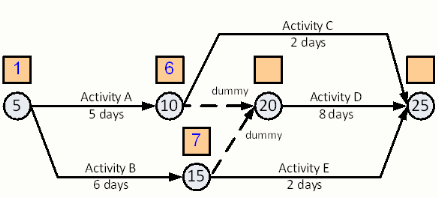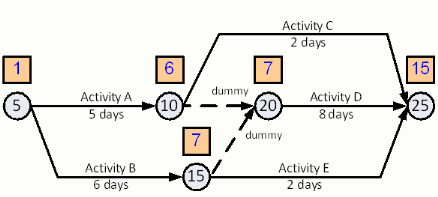# dummies

Since any real Arrow Diagram can be expected to include logic and numbering dummies, lets do an example now with an Arrow Diagram that has logic dummies. The example project is shown below as five work activities and two dummy activities.Given the standard project start date of day one (1), the Early Event Times at node-10 and node-15 are easy to calculate. Now we have dummies between nodes 10-20 and 15-20 to calculate. The first thing to note is that dummy activities have, by definition, zero duration. Given that we can calculate the Early Event Time for node-20 just like any other activity with multiple incoming activities. The selection of the Early Event Time for a given node is the latest value at the end of any of the incoming activities. Since the Early Event Time from node-15 plus the duration of the dummy activity from node-15 to node-20, i.e. zero (0), is later than the Early Event Time provided from the dummy at node-10 to node-20, the Early Even Time at node-20 is 7.Once we know the Early Event Time of node-20 we can calculate the Early Event Time at node-25. The latest of each of the incoming Early Event Times is 15. This value comes from the Early Event Time from node-20 plus the activity duration of Activity D.The longest two activities in the network are Activity B and Activity D. These activities govern the length of the whole project. It is interesting to note that the longest path through the network from node-5, to node-15, to node-20, to node-25 contains a dummy activity with zero duration!